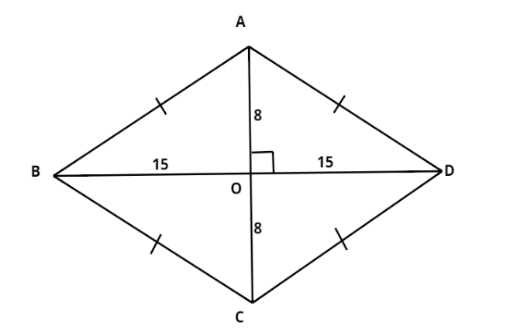QuestionAnswers

# The diagonals of rhombus measure 16cm and 30cm. Find its perimeter.Hint – Here first we draw a rhombus after that to determine its perimeter we will use the concept that Diagonals of rhombus bisect perpendicular to each other and Perimeter of rhombus $\;4 \times side$.AC and BD are diagonals of a rhombus.
AC=16, BD=30
We know that diagonals of rhombus are perpendicular bisectors of each other.
$\therefore$ AO = 8 and BO = 15
In right angled $\Delta AOB$
$\begin{array}{*{20}{l}} { \Rightarrow \;\;{{\left( {AB} \right)}^2} = {{\left( {AO} \right)}^2} + {{\left( {BO} \right)}^2}} \\ { \Rightarrow \;\;{{\left( {AB} \right)}^2} = {{\left( 8 \right)}^2} + {{\left( {15} \right)}^2}} \\ { \Rightarrow \;\;{{\left( {AB} \right)}^2} = 64 + 225} \\ { \Rightarrow \;\;{{\left( {AB} \right)}^2} = 289} \\ { \Rightarrow \;\;AB = 17cm} \end{array}$
We know that all four sides of rhombus are equal.
$\therefore$ Perimeter of rhombus ABCD $= {\text{ }}4 \times AB$
$\begin{array}{*{20}{l}} {\; = {\text{ }}4 \times 17} \\ { = {\text{ }}68cm} \end{array}$

Note – In this problem, first we calculate the side of the rhombus with help of Pythagoras theorem then calculate the perimeter of the rhombus by using the concept which is given above.
View Notes
Area of a Rhombus FormulaTo Measure the Volume of an Irregular Lamina Using Screw GaugeCBSE Class 9 Maths Chapter 6 - Lines and Angles FormulasCBSE Class 9 Maths Chapter 13 - Surface Areas and Volumes FormulasCBSE Class 9 Maths FormulasTable of 9 - Multiplication Table of 9CBSE Class 8 Maths Chapter 9 - Algebraic Expressions and Identities FormulasCBSE Class 9 Maths Chapter 15 - Probability FormulasCBSE Class 9 Maths Chapter 2 - Polynomials FormulasCBSE Class 7 Maths Chapter 9 - Rational Numbers FormulasImportant Questions for CBSE Class 9 Maths Chapter 9 - Areas of Parallelograms and TrianglesImportant Questions for CBSE Class 9 Science Chapter 4 - Structure of The AtomImportant Questions for CBSE Class 9 Science Chapter 9 - Force and Laws of MotionImportant Questions for CBSE Class 9 Science Chapter 5 - The Fundamental Unit of LifeNCERT Books for Class 9 Maths Chapter 9Important Questions for CBSE Class 9 MathsImportant Questions for CBSE Class 6 Science Chapter 9 - The Living Organisms and Their SurroundingsImportant Questions for CBSE Class 9 Maths Chapter 6 - Lines and AnglesImportant Questions for CBSE Class 10 Maths Chapter 9 - Some Applications of TrigonometryImportant Questions for CBSE Class 8 Social Science Our Past 3 Chapter 9 - The Making of the National Movement: 1870s -1947CBSE Class 12 Maths Question Paper 2020Previous Year Question Paper of CBSE Class 10 EnglishCBSE Class 10 Maths Question Paper 2020Maths Question Paper for CBSE Class 10 - 2011Maths Question Paper for CBSE Class 10 - 2008CBSE Class 10 Maths Question Paper 2017Maths Question Paper for CBSE Class 10 - 2012Maths Question Paper for CBSE Class 10 - 2009Maths Question Paper for CBSE Class 10 - 2010Maths Question Paper for CBSE Class 10 - 2007NCERT Solutions for Class 9 Maths Chapter 9 Areas of Parallelograms and TrianglesNCERT Solutions For Class 9 Science Chapter 9RD Sharma Solutions for Class 9 Maths Chapter 9 - Triangle and its AnglesRD Sharma Class 9 Solutions Chapter 9 -Triangle and Its Angles (Ex 9.2) Exercise 9.2RD Sharma Class 9 Solutions Chapter 9 -Triangle and Its Angles (Ex 9.1) Exercise 9.1NCERT Solutions for Class 9 Maths Chapter 9 Areas of Parallelograms and Triangles (Ex 9.2) Exercise 9.2NCERT Solutions for Class 9 Maths Chapter 6 Lines and AnglesNCERT Solutions for Class 11 Maths Chapter 9 Sequences and SeriesNCERT Solutions for Class 9 Maths Chapter 9 Areas of Parallelograms and Triangles (Ex 9.1) Exercise 9.1NCERT Solutions for Class 9 Maths Chapter 9 Areas of Parallelograms and Triangles (Ex 9.4) Exercise 9.4